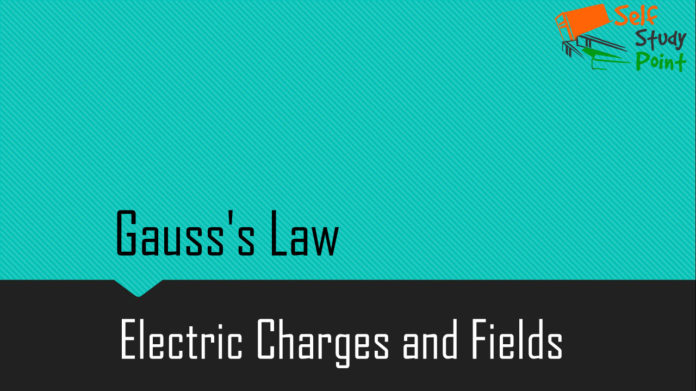The surface integral of electrostatic field produced by any source over any closed surface S enclosing a volume V in vacuum i.e. total electric flux over the closed surface S in vacuum is 1/epsilon times the total charge Q contained inside S.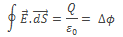The surface chosen to calculate the surface integral is called Gaussian surface.

## Proof of Gaussian Theorem

The flux through area element ∆S is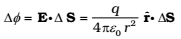The unit vector rˆ is along the radius vector from the centre to the area element. Now, since the normal to a sphere at every point is along the radius vector at that point, the area element ΔS and rˆ have the same direction. Therefore,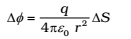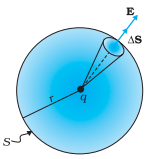Since the magnitude of a unit vector is 1. The total flux through the sphere is obtained by adding up flux through all the different area elements:Since each area element of the sphere is at the same distance r from the charge,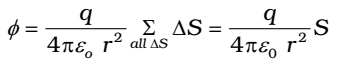Now S, the total area of the sphere, equals 4πr2 . Thus,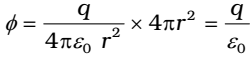### SIGNIFICANCE OF GAUSS’S LAW

1. Gauss’s law is true for any closed surface, no matter what its shape or size.
2. The term q on the right side of Gauss’s law includes the sum of all charges enclosed by the surface. The charges may be located anywhere inside the surface.
3. In the situation when the surface is so chosen that there are some charges inside and some outside, the electric field is due to all the charges, both inside and outside S. The term q on the right side of Gauss’s law.
4. Gauss’s law is often useful towards a much easier calculation of the electrostatic field when the system has some symmetry. This is facilitated by the choice of a suitable Gaussian surface.
5. Finally, Gauss’s law is based on the inverse square dependence on distance contained in the Coulomb’s law. Any violation of Gauss’s law will indicate departure from the inverse square law.